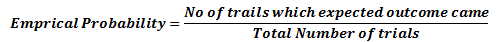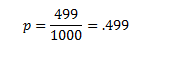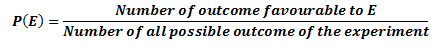# Class 10 Maths notes for Probability

Table of Content

## What is Probability ?

Probability is the measure of the likeliness that an event will occur. Probability is quantified as a number between 0 and 1 (where 0 indicates impossibility and 1 indicates certainty)

## Why we need Probability and what is the use of it?

It is widely used in the study of Mathematics, Statistics, Gambling, Physical sciences, Biological sciences, Weather forecasting, Finance etc. to draw conclusions. Insurance companies uses this to decide on financial policies

## There are few terms related to Probability which are defined below

### Randomness

In mathematics, When next outcome of the experiment cannot be determined then we say it is a random experiment e.g. Consider the dice. When we throw the dice, we cannot determine what number will come Since we cannot predict the next outcome, we may say it is a random experiment

### Trial

A trial is an action which results in one or several outcomes, for example each toss of the coin and each throw of the die are called trials

### Independent Trial

:Successive trials of some random event for example tosses of a coin,throws of a die are said to be independent if the outcome of any one trial does not impact the outcomes of any others.

### Experiment

Experiment and trial are same thing as such.An experiment is a situation involving chance or probability that leads to results called outcomes.But sometimes we use experiment to refer to whole large number of trials.

### Event

An event is a possible outcome of the Experiment. Like head coming in a toss

### Sample space

It is a set of all possible outcomes of an experiment.

e.g. when we coin is tossed,the possible outcome are Head and Tail.So sample space is Head and tail

## Empirical Probability:

1) Experimental or empirical probability is an estimate that an event will happen based on how often the event occurs after performing an experiment in a large number of trials.
2) It is a probability of event which is calculated based on experimentsExample:
A coin is tossed 1000 times, we get 499 times head and 501 times tail,
So empirical or experimental probability of getting head is calculated asEmpirical probability depends on experiment and different will get different values based on the experiment

## Theoretical Probability

The theoretical probability or the classical probability of the event is defined asSome Important points
1. Here in this probability approach, it is assumed that all the events of the experiment are equally likely
2. An event having only one outcome of the experiment is called an elementary event.
The sum of the probabilities of all the elementary events of an experiment is 1.”
i.e. If we three elementar event A,B,C in  the experiment ,then
$P(A)+P(B) +P(C)=1$
3. The event $\bar{A}$, representing ‘not A’, is called the complement of the event A. We also say that $\bar{A}$ and A are complementary events. Also
$P(A) + P(\bar{A}=1$
4. The probability of an event (U) which is impossible to occur is 0. Such an event is called an impossible event
$P(U)=0$
5. The probability of an event ( X) which is sure (or certain) to occur is 1. Such an event is called a sure event or a certain event
$P(X)=1$
6. Probability of any event can be as
$0 \leq p \leq 1$
Question 1
A dice is thrown once. What is the probability of getting a number greater than 4?
Solution
Total possible outcome=6
Favourable outcome (5,6) =2
Probability = 2/6= 1/3

Question 2
Cards with numbers 2 to 101 are placed in a box. A card selected at random from the box. Find the probability that the card which is selected has a number which is a perfect square.
Solution
Total number=100
Favourable outcome ( 4,9,16,25,36,49,64,81,100) =9
Probability = $\frac {9}{100}$

Question 3
A card is drawn from a well shuffled deck of 52 cards. Find the probability of getting an ace.
Solution
Total number=52
Favourable outcome =4
Probability = $\frac { 4}{52}=\frac {1}{13}$

Question 4
Find the probability that a leap year selected randomly will have 53 Sundays?
Solution
No. of days in a leap year = 366 days = 52 weeks + 2 days
It implies a leap year will have 52 Sundays. In remaining 2 days, possible outcomes are:
Sun, Mon
on, Tue
Tue, Wed
Wed, Thu
Thu, Fri
Fri, Sat
Sat, Sun
Total out comes = 7 Favourable outcomes that Sunday will come in these two days = 2
Required probability = $\frac {2}{7}$

## Brain Teaser

Solve these Jumbled word for the main keywords in this chapter
Example AESPLM -> SAMPLE
• BLBTPIYRIOA
• ARITL
• LMCERPIA
• TAEOHTCRIEL
• SSLOBPIMIE
• VENET
• RMCNPMMPEOYAETL
• EMAREYLNTE

• probability
• trial
• emprical
• Theoretical
• impossible
• event
• complementary
• elementary

### Quiz Time

Question 1
The probability of the sure events is
A. 0
B. 1
C. .5
D. -1
Question 2
Which is of these cannot be the probability of the event ?
A. 1.1
B. .5
C. 0
D. 1
Question 3
The sample space of a throw of two coin is
A. { (H,H),(T,T)}
B. { (H,H),(T,T),(H,T),(T,H)}
C. { (H,T),(T,H)}
D. {H,T}
Question 4
A card is drawn from a well shuffled deck of 52 cards. Find the probability of getting an Queen
A. $\frac {1}{12}$
B. $\frac {2}{13}$
C. $\frac {1}{13}$
D. 1
Question 5
Cards with alphabets A to Z placed in a box. A card selected at random from the box. Find the probability that the card which is selected has a vowel?
A. $\frac {1}{26}$
B. $\frac {5}{26}$
C. $\frac {1}{13}$
D. $\frac {2}{13}$
Question 6
if $P(A) =.25$, then $P(A^c) \; is$
A. 1.25
B. .50
C. .75
D. 0Go back to Class 10 Main Page using below links

### Practice Question

Question 1 What is $1 - \sqrt {3}$ ?
A) Non terminating repeating
B) Non terminating non repeating
C) Terminating
D) None of the above
Question 2 The volume of the largest right circular cone that can be cut out from a cube of edge 4.2 cm is?
A) 19.4 cm3
B) 12 cm3
C) 78.6 cm3
D) 58.2 cm3
Question 3 The sum of the first three terms of an AP is 33. If the product of the first and the third term exceeds the second term by 29, the AP is ?
A) 2 ,21,11
B) 1,10,19
C) -1 ,8,17
D) 2 ,11,20